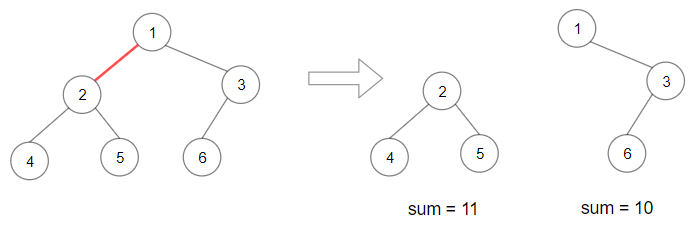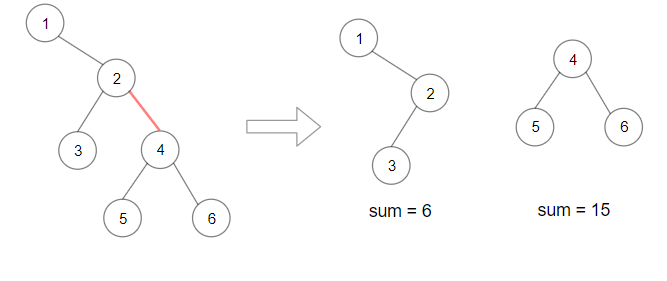Tags: "leetcode", "dynamic-programming", access_time 1-min read

# Maximum Product of Splitted Binary Tree

#### Created: April 2, 2020 by [lek-tin]

##### Last updated: April 2, 2020

Given a binary tree root. Split the binary tree into two subtrees by removing 1 edge such that the product of the sums of the subtrees are maximized.

Since the answer may be too large, return it modulo `10e9 + 7`.

### Example 1``````Input: root = [1,2,3,4,5,6]
Output: 110
Explanation: Remove the red edge and get 2 binary trees with sum 11 and 10. Their product is 110 (11*10)
``````

### Example 2``````Input: root = [1,null,2,3,4,null,null,5,6]
Output: 90
Explanation:  Remove the red edge and get 2 binary trees with sum 15 and 6.Their product is 90 (15*6)
``````

### Example 3

``````Input: root = [2,3,9,10,7,8,6,5,4,11,1]
Output: 1025
``````

### Example 4

``````Input: root = [1,1]
Output: 1
``````

Constraints:

1. Each tree has at most 50000 nodes and at least `2` nodes.
2. Each node’s value is between [`1, 10000]`.

### Solution

``````# Definition for a binary tree node.
# class TreeNode:
#     def __init__(self, x):
#         self.val = x
#         self.left = None
#         self.right = None

class Solution:
def maxProduct(self, root: TreeNode) -> int:
MOD = 10**9 + 7
total = self.dfs(root)
self.maxProd = 0
self.dfs(root, total)
return self.maxProd % MOD

def dfs(self, root, total=None):
if not root:
return 0
subtotal = self.dfs(root.left, total) + self.dfs(root.right, total)+root.val
if total != None:
print(total, self.maxProd)
self.maxProd = max(self.maxProd, subtotal*(total-subtotal))
return subtotal
``````